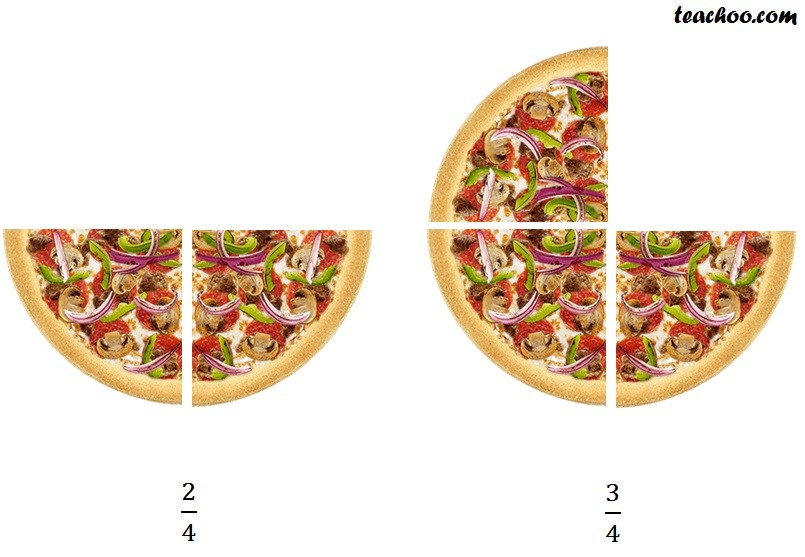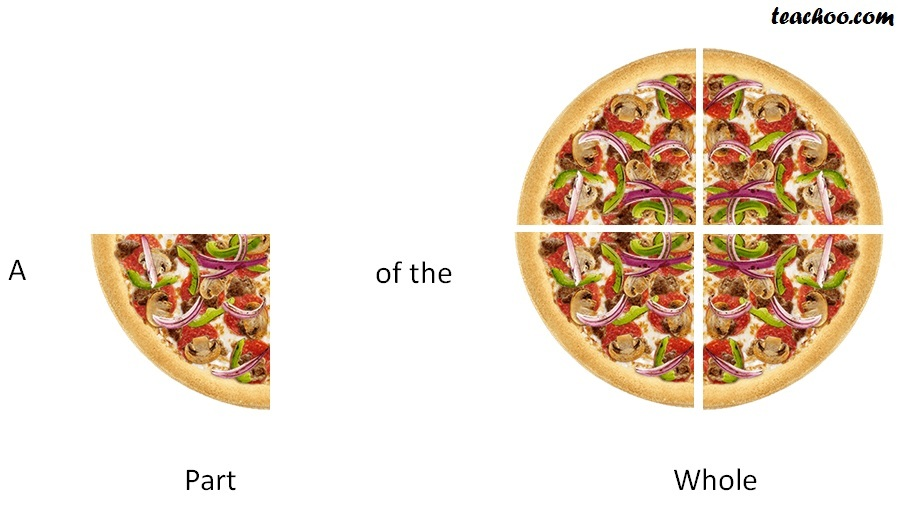Suppose I have a PizzaAnd I divide it into 4 parts

Then, how much isIt is 1 part of 4 parts

So, we write it as, 1/4

We pronounce it as, One by four.

Similarly,This   1/4,  2/4,  3/4 is called fractions

Fraction are the part of the whole.1. Chapter 7 Class 6 Fractions
2. Concept wise
3. Definitions

Definitions

Chapter 7 Class 6 Fractions
Concept wise